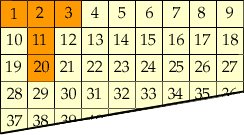Look at the T-shape drawn on the 9 by 9 number grid. The total of the numbers inside the T-shape is 1 + 2 + 3 + 11 + 20 = 37. This is called the T-total The number at the bottom of the T-shape is called the T-number. The T-number of this T-shape is 20. Translate the T-shape to different positions on the grid. Investigate the relationship between the T-total and the T-number. Mr M.WisemanHi,

Suppose that you place the T-shape on the grid in some position. Suppose that the T-number is N. The number in the T, directly above the T-number is then 9 places back in the grid so it is N - 9. The number directly above this number is then N - 9 -9 = N - 18. The two remaining numbers in the T-shape are N - 18 + 1 and N - 18 - 1. Thus the T-total is

N + (N - 9) + (N - 18) + (N - 18 + 1) + (N - 18 - 1)
= 5N - 63

Cheers,
Penny
Go to Math Central